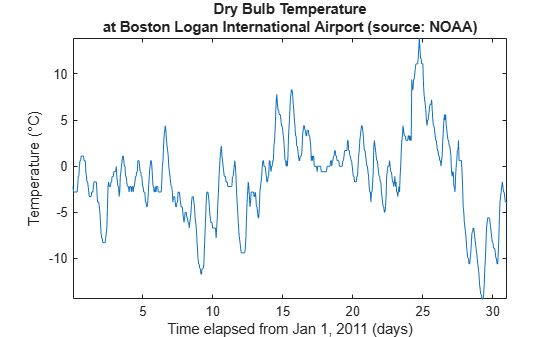# extract

Extract time-domain or frequency-domain features

## Syntax

``features = extract(sFE,x)``
``[features,info] = extract(sFE,x)``
``[features,info,framelimits] = extract(sFE,x)``

## Description

example

````features = extract(sFE,x)` returns a matrix or a table containing features extracted from input `x`. The output depends on the settings of the feature extractor object `sFE`.```

example

````[features,info] = extract(sFE,x)` returns a structure `info` that maps a specific feature to its column location in the output feature matrix `features`. This syntax is valid only when you set the `FeatureFormat` property of the feature extractor object to `"matrix"`.```
````[features,info,framelimits] = extract(sFE,x)` returns a matrix `framelimits` whose i-th row contains the beginning and end limits of the i-th frame. This syntax is valid only when you set the `FeatureFormat` property of the feature extractor object to `"matrix"`.```

## Examples

collapse all

Load a set of temperature readings in Celsius taken every hour at Logan Airport in Boston for 31 days. Plot the data.

```load bostemp days = (1:31*24)/24; plot(days, tempC) axis tight ylabel('Temp (\circC)') xlabel('Time elapsed from Jan 1, 2011 (days)') title('Logan Airport Dry Bulb Temperature (source: NOAA)')```Create a `signalTimeFeatureExtractor` object and enable the `PeakValue` feature. To obtain the maximum absolute temperature reading per day, set the frame size to `24` samples and the frame overlap to `0` samples.

`sFE = signalTimeFeatureExtractor(FrameSize=24,FrameOverlapLength=0,PeakValue=true);`

Call the `extract` function on the object to extract the daily absolute maximum temperatures in the data set.

`peaktemps = extract(sFE,tempC)`
```peaktemps = 31×1 2.8000 6.1000 8.3000 3.3000 2.8000 4.4000 4.4000 6.1000 10.6000 11.7000 ⋮ ```

Confirm the extracted peak values. Divide the signal into 24-sample segments representing temperature readings per day and compute the maximum absolute value of each segment. Compare the resulting vector to `peaktemps`.

```y = buffer(tempC,24); [mx,idx] = max(abs(y)); tf = isequal(peaktemps,mx')```
```tf = logical 1 ```

Consider a quadratic chirp sampled at 1 kHz for 2 seconds. The chirp has an initial frequency of 100 Hz that increases to 200 Hz at t = 1 second. Compute and display the spectrogram.

```fs = 1e3; t = 0:1/fs:2; y = chirp(t,100,1,200,'quadratic'); pspectrum(y,fs,'spectrogram')```Create a `signalFrequencyFeatureExtractor` object to obtain the mean and median frequencies from the signal. Specify the sample rate.

`sFE = signalFrequencyFeatureExtractor(SampleRate=fs,MeanFrequency=true,MedianFrequency=true);`

Extract the features. `info` returns the column index in `features` of each extracted feature.

`[features,info] = extract(sFE,y)`
```features = 1×2 226.0160 199.7034 ```
```info = struct with fields: MeanFrequency: 1 MedianFrequency: 2 ```

Set the `FrameSize` and `FrameRate` properties of the feature extractor object to divide the signal into two frames. The first frame represents the chirp oscillating at the initial frequency of 100 Hz and the second frame represents the chirp oscillating at 200 Hz. Extract the mean and median frequencies for each frame and include the frame limits in the output.

```sFE.FrameSize = round(length(y)/2); sFE.FrameRate = 1000; [features,info,framelimits] = extract(sFE,y)```
```features = 2×2 131.4921 124.9820 331.2664 324.6992 ```
```info = struct with fields: MeanFrequency: 1 MedianFrequency: 2 ```
```framelimits = 2×2 1 1001 1001 2001 ```

## Input Arguments

collapse all

Feature extractor object, specified as a `signalFrequencyFeatureExtractor` object or a `signalTimeFeatureExtractor` object.

Input signal, specified as a vector or matrix.

Data Types: `single` | `double`

## Output Arguments

collapse all

Extracted features, returned as an L-by-M-by-N array:

• L — Number of frames

• M — Number of features extracted per frame

• N — Number of channels

When you set the `FeatureFormat` property of the input feature extractor object to `"table"`, the function returns the extracted features in a table with the frame limits listed in the first two table variables. When you set the `FeatureFormat` property of the input feature extractor object to `"matrix"`, the function returns the extracted features in a matrix.

Feature information, returned as a structure. The function maps each feature to its column location in the output matrix `features`. This argument applies only when you set the `FeatureFormat` property of the input feature extractor object to `"matrix"`.

Frame limits, returned as a matrix. The i-th row in `framelimits` contains the beginning and end limits of the i-th frame. This argument applies only when you set the `FeatureFormat` property of the input feature extractor object to `"matrix"`.

## Version History

Introduced in R2021b